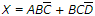# Digital Electronics - Combinational Logic Circuits - Discussion

### Discussion :: Combinational Logic Circuits - True or False (Q.No.34)

34.is in the form of a sum-of-products expression.

 [A]. True [B]. False

Explanation:

No answer description available for this question.

 Manas said: (Sep 23, 2011) In a SOP all the variables must be present in the expression(either in true or complement form). so the answer will be false. here in the 1st term D is missing so multiply it with D+D' and similarly in the 2nd term multiply with A+A'. the sop will be ABC'D + ABC'D'+ ABCD'+ A'BCD'